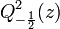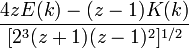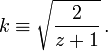User:Tohline/Math/EQ Q2minusHalf01$~Q^2_{-\frac{1}{2}}(z)$$~=$$~ \frac{ 4 z E(k) - (z-1) K(k) }{ [2^{3} (z+1) (z-1)^{2} ]^{1 / 2}}$ where:$~k \equiv \sqrt{ \frac{2}{z+1}} \, .$ (see our associated derivation)